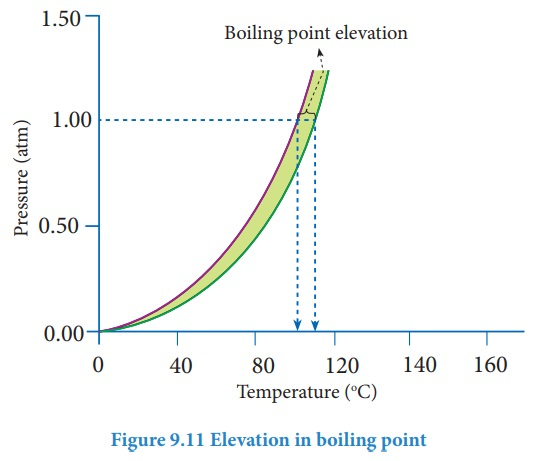Home | | Chemistry 11th std | Elevation of boiling point

# Elevation of boiling point

Boiling point is an important physical property of a liquid.

Elevation of boiling point

Boiling point is an important physical property of a liquid. The boiling point of a liquid is the temperature at which its vapour pressure becomes equal to the atmospheric pressure (1 atm). When a nonvolatile solute is added to a pure solvent at its boiling point, the vapour pressure of the solution is lowered below 1 atm. To bring the vapour pressure again to 1 atm, the temperature of the solution has to be increased. As a result,the solution boils at a higher temperature (Tb) than the boiling point of the pure solvent (Tb┬░). This increase in the boiling point is known as elevation of boiling point. A plot of vapour pressure versus temperature for water and an aqueous solution is given belowThe vapour pressure of the solution increases with increase in temperature as shown in the above figure. The variation of vapour pressure with respect to temperature for pure water is given by the violet coloured curve. At 100 Ōü░C the vapour pressure of water is equal to 1 atm. Hence the boiling point of water is 100 Ōü░C (Tb┬░). When a solute is added to water, the vapour pressure of the resultant solution is lowered. The variation of vapour pressure with respect to temperature for the solution is given by green curve. From the graph, it evident the vapour pressure of the solution is equal to 1 atm pressure at the temperature Tb which is greater than Tb┬░. The difference between these two temperatures (Tb-Tb┬░) gives the elevation of boiling point.

The elevation of boiling point (╬öTb)= Tb - Tb┬░

The elevation of boiling point is directly proportional to the concentration of the solute particles.

╬öTb ╬▒ m (9.23)

m is the concentration of solution expressed in molality.

╬öTb = Kb m (9.24)

Where

Kb = molal boiling point elevation constant or Ebullioscopic constant.

If m=1, then ╬öTb=Kb;

Hence, the Kb is equal to the elevation in boiling point for 1 molal solution. Kb is calculated by the following expression## Problem:

0.75 g of an unknown substance is dissolved in 200 g water. If the elevation of boiling point is 0.15 K and molal elevation constant is 7.5 K Kg mol-1 then, calculate the molar mass of unknown substance

╬öTb  = Kb m

= Kb x W2 x 1000 / M2 x W1

M2 = Kb x W2 x 1000 / ╬öTb x W1

= 7.5 x 0.75 x 1000 / 0.15 x 200

= 187.5 g mol-1

Study Material, Lecturing Notes, Assignment, Reference, Wiki description explanation, brief detail
11th Chemistry : UNIT 9 : Solutions : Elevation of boiling point |